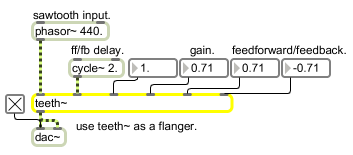teeth~

Comb filter with feedforward and feedback delay control

Description

The teeth~ object implements a comb filter, in which a slightly delayed version of a signal is added to itself, causing phase cancellations and a spectrum that looks like a comb. The teeth~ object is a variation of the comb~ object and implements a similar filter formula:

y[n] = a * x[n] + b * x[n - FFdelay] + c * y[n - FBdelay]

Arguments

Name Type Opt Description
feedforward/feedback-delays gain-coefficient and feedforward/feedback-gain-coefficients float opt Up to six numbers, to set the feedforward and feedback delays, the gain coefficient, and the feedforward and feedback gain coefficients. If a signal is connected to a given inlet, the coefficient supplied as an argument for that inlet is ignored. If no arguments are present, the maximum delay time defaults to 10 milliseconds, and all other values default to 0.

Messages

 int filter-parameters [int] The filter parameters in inlets 2 to 6 may be specified by a float instead of a signal. If a signal is also connected to the inlet, the float is ignored. float filter-parameters [float] The filter parameters in inlets 2 to 6 may be specified by a float instead of a signal. If a signal is also connected to the inlet, the float is ignored. clear Clears the teeth~ object's memory of previous outputs, resetting them to 0. signal In left inlet: Signal to be filtered. The teeth~ object is a variant of comb~-- a comb filter that mixes the current input sample with earlier input and/or output samples to accentuate and attenuate the input signal at regularly spaced frequency intervals. Unlike the comb~ object, teeth~ adds feedforward and feedback, which adds to the extremity of the effect. In 2nd inlet: Feedforward - the delay, in milliseconds, before past samples of the input are added to the current input. In 3rd inlet: Feedback - the delay, in milliseconds, before past samples of the output are added to the current input. In 4th inlet: Gain coefficient - for scaling the amount of the input sample to be sent to the output. In 5th inlet: Gain coefficient - for scaling the amount of feedforward to be sent to the output. In right inlet: Gain coefficient - for scaling the amount of feedback to be sent to the output. list filter-parameters [list] The six parameters can be provided as a list in the left inlet. The first number in the list is the feedforward delay, the next number is the feedback delay, the third number is the Gain coefficient for the input sample, the fourth number is the feedforward gain coefficient, and the fifth number is the feedback gain coefficient. If a signal is connected to a given inlet, the coefficient supplied in the list for that inlet is ignored.

Output

signal: The filtered signal.

Examplesteeth~ does comb filtering on an input signal with variable feedforward and feedback delays